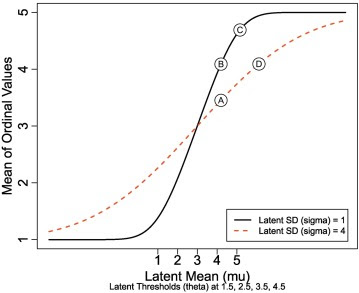## Friday, September 7, 2018

### Analyzing ordinal data with metric models: What could possibly go wrong? (just published)

Just published:

Analyzing ordinal data with metric models: What could possibly go wrong?
We surveyed all articles in the Journal of Personality and Social Psychology(JPSP), Psychological Science (PS), and the Journal of Experimental Psychology: General (JEP:G) that mentioned the term “Likert,” and found that 100% of the articles that analyzed ordinal data did so using a metric model. We present novel evidence that analyzing ordinal data as if they were metric can systematically lead to errors. We demonstrate false alarms (i.e., detecting an effect where none exists, Type I errors) and failures to detect effects (i.e., loss of power, Type II errors). We demonstrate systematic inversions of effects, for which treating ordinal data as metric indicates the opposite ordering of means than the true ordering of means. We show the same problems — false alarms, misses, and inversions — for interactions in factorial designs and for trend analyses in regression. We demonstrate that averaging across multiple ordinal measurements does not solve or even ameliorate these problems. A central contribution is a graphical explanation of how and when the misrepresentations occur. Moreover, we point out that there is no sure-fire way to detect these problems by treating the ordinal values as metric, and instead we advocate use of ordered-probit models (or similar) because they will better describe the data. Finally, although frequentist approaches to some ordered-probit models are available, we use Bayesian methods because of their flexibility in specifying models and their richness and accuracy in providing parameter estimates. An R script is provided for running an analysis that compares ordered-probit and metric models.Fig. 4. Ordinal mean as a function of latent mean (mu) and SD (sigma). Groups marked Ⓐ and Ⓑ illustrate a false alarm (Type I error) for which the underlying means are exactly equal but the ordinal means are very different. Groups marked Ⓑ and Ⓓ illustrate a miss (Type II error) for which the underlying means are quite different but the ordinal means are exactly equal. Groups marked Ⓒ and Ⓓ illustrate an inversion for which the underlying means have μD > μC but the ordinal means incorrectly have μC > μD.

Published article:
https://www.sciencedirect.com/science/article/pii/S0022103117307746

Preprint manuscript: https://osf.io/9h3et/

R code: https://osf.io/53ce9/files/